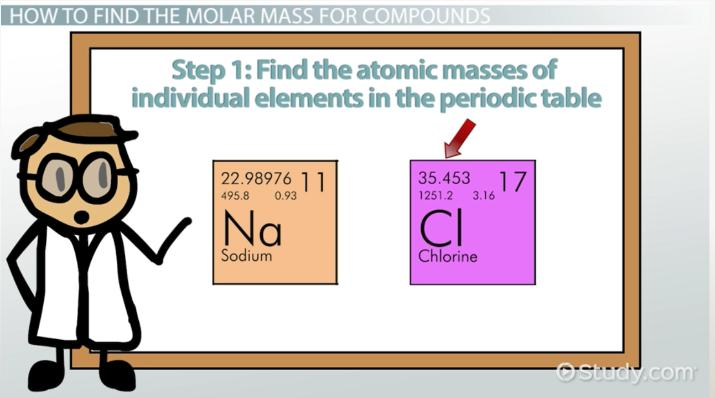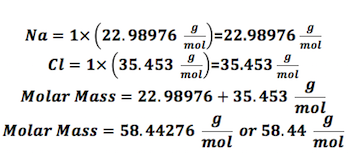Science Courses / Course / Chapter

# What is Molar Mass? - Definition, Formula & Examples

Lesson Transcript
Instructor
Nissa Garcia

Nissa has a masters degree in chemistry and has taught high school science and college level chemistry.

Expert Contributor
Matthew Bergstresser

Matthew has a Master of Arts degree in Physics Education. He has taught high school chemistry and physics for 14 years.

Molar mass is a unit of calculation that describes the sum of the mass of a substance per mole. Learn the formula used to calculate the molar mass of substances, and understand its usefulness through an example problem. Updated: 10/26/2021

## What is Molar Mass?

Substances take up space and have mass. Molecules, which make up substances, often need to be measured in experiments, and it is important that these measurements are accurate. But, how can we measure something so small in an accurate way? How do we normally measure molecules? In the science laboratory, we use a tool called an analytical balance to measure in grams.

Through careful calculation, scientists can determine the number of moles a specific reaction will need. A mole is a unit of measure that helps us compare particles of any given substance and its mass. If we already know the number of moles needed, we can use the concept of molar mass to calculate how many grams of the substance are required. The molar mass, also known as molecular weight, is the sum of the total mass in grams of all the atoms that make up a mole of a particular molecule. The unit used to measure is grams per mole.An error occurred trying to load this video.

Try refreshing the page, or contact customer support.

Coming up next: What is Nickel? - Definition & Explanation

### You're on a roll. Keep up the good work!

Replay
Your next lesson will play in 10 seconds
• 0:01 What Is Molar Mass?
• 1:00 Finding Molar Mass for…
• 2:56 Calculating Molar Mass…
• 4:18 Calculating # of Grams…
• 5:34 Lesson Summary
Save Timeline
Autoplay
Autoplay
Speed Speed## How to Find the Molar Mass for Compounds

Compounds are substances that are made up of more than one element. For example, some common compounds include salt, glucose, acetic acid (or vinegar), and sodium bicarbonate (or baking soda).

The compound sodium chloride is made up of two elements, namely, sodium and chlorine. Let's use this compound, sodium chloride, as our first example on how to calculate the molar mass for the compounds. The first thing we need to do is to find sodium and chlorine in the periodic table.

### Step 1: Find the atomic masses of individual elements in the periodic table

The first thing we need is to find the individual atomic masses for each element. If you look at the entries for sodium and chlorine in the table, we will pay attention to the numbers that appear in the top left (see video). These represent the atomic mass. The element sodium has an atomic mass of 22.98976 g/mol. The element chlorine has an atomic mass of 35.453 g/mol.

### Step 2: Count how many atoms there are for each element

For the compound sodium chloride, since there are no subscripts (small numbers at the bottom of each element's symbol), that means there is only one sodium and only one chlorine atom for this compound.

### Step 3: Find the molar mass

Now that we know how many atoms there are for each element, we can find the molar mass.First, we calculate the mass of the sodium atoms, which is 22.98976 grams per mole. Next, we do the same for the mass of chlorine atoms, which is 35.453 grams per mole. Then, we add these two masses together to find the total mass of sodium chloride molecules. This comes out to 58.44276 grams per mole which we can round to 58.44 grams per mole.

## Example: Calculating the Molar Mass of a Compound

Let's look at a more complicated example.

Find the molar mass of acetic acid.

There are three elements - carbon, hydrogen and oxygen - so find their atomic masses in the periodic table: 12.0107 grams per mol for carbon, 1.00794 grams per mol for hydrogen, and 15.9994 grams per mol for oxygen.

Next, we must consider that each element in the chemical compound has a subscript, so there are more than one of each atom. We have two carbon atoms, four hydrogen atoms, and two oxygen atoms.

To unlock this lesson you must be a Study.com Member.

## Molar Mass

Molar mass is the mass equivalent of Avogadro's number of atoms of an element, or Avogadro's number of molecules of in a chemical compound. Avogadro's number is 6.02 x 1023 atoms per mole or molecules per mole. The periodic table lists the molar mass of each element. Let's practice determining the molar mass of some common substances. You will need access to a periodic table to complete these calculations. A calculator will also be useful.

### Problems for More Practice

1. Determine the molar mass of silver.

2. Determine the molar mass of gold.

3. Determine the molar mass of sodium oxide (Na2 O).

4. Determine the molar mass of calcium sulfide (CaS).

5. Determine the molar mass of ozone (O3).

### Solutions

1. The molar mass of silver is 107.87 g/mol.

2. The molar mass of gold is 196.97 g/mol.

3. The molar mass of sodium oxide is 61.98 g/mol: 2(Na) + 1(O) = 22.99 g/mol + 16 g/mol = 61.98 g/mol.

4. The molar mass of calcium sulfide is 72.14 g/mol: 1(Ca) + 1(S) = 40.08 g/mol + 32.06 g/mol = 72.14 g/mol.

5. The molar mass of ozone is 48 g/mol: (3)(16 g/mol) = 48 g/mol.

### Register to view this lesson

Are you a student or a teacher?

Back

### Resources created by teachers for teachers

Over 30,000 video lessons & teaching resources‐all in one place.Video lessonsQuizzes & WorksheetsClassroom IntegrationLesson Plans

I would definitely recommend Study.com to my colleagues. It’s like a teacher waved a magic wand and did the work for me. I feel like it’s a lifeline.

Jennifer B.
TeacherCreate an account to start this course today
Used by over 30 million students worldwide